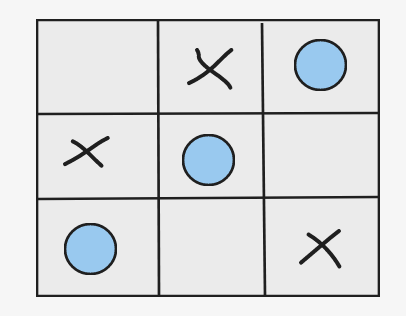# Winner of Tic-Tac-Toe

## Introduction

Tic-Tac-Toe - the game that we have all played multiple times in between or during classes scribbling away on the back of notebooks coming up with strategies and arguing over who goes first. Well, all that somehow did pay off cause the Determination of the Winner of Tic-Tac-Toe is a common interview problem. The rules are the same as they have always been just that instead of a scribble board or a notebook, here we have a matrix to place our moves into. Sounds simple enough right well it is simple and let's get right to it.

(Also see Data Structures)

## Problem Statement

Tic-tac-toe is a grid-based game played by two players, A and BZ, on a 3 x 3 grid.

The rules of Tic-Tac-Toe are:

1. Players take turns placing characters into empty squares' '.
2. The first player A always places 'X' characters, while the second player B always places 'O' characters.
3. 'X' and 'O' characters are always placed into empty squares, never on filled ones.
4. The game ends when three of the same (non-empty) characters fill any column, row, diagonal, or if all squares are non-empty.
5. If the game is over, no more moves can be played.

Given a 2D integer array moves where moves[i] = [row-i, col-i] indicates that the i-th move will be played on grid[row-i][col-i], return the winner(A or B) of the game if it exists.

Return "Pending" if there are still movements to play and return "Draw" if the game ends in a draw.

You can assume that the move is valid (i.e., it follows the rules of Tic-Tac-Toe), the grid is initially empty, and A will play first

Example

Input: moves = [[2,2],[1,1],[0,1],[0,2],[1,0],[2,0]]

Output: "B" as the B wins if drawn a diagram for the same.Let's get started and learn the approach to solve this problem.

Recommended: Before moving on to the solution, try the Problem yourself first.

## Approach

There are 8 ways to win Tic-tac-toe for a player, i.e., 3 columns, 3 rows, and 2 diagonals. Both A and B players make a move alternatively. So, all the odd moves are for player A and even moves for player B.

Now, to track the movement of the players and if the winning positions are filled up, an array for each player is maintained.

Each index in the player’s array holds a specific meaning:

0,1,2 index for rows

3,4,5 index for columns

6 index for diagonal (top left to bottom right)

7 index for diagonal (top right to bottom left)

0    1    2  columns

0   [ ]  [ ]  [ ]

1   [ ]  [ ]  [ ]

2   [ ]  [ ]  [ ]

Rows

So,  if move made at row 0, 1 or 2  ----> player[row] is updated

if move made at column 0,1 or 2 ----> player[column + 3] is updated

If move made at diagonal (top left to bottom right) ({0,0}, {1,1}, {2,2}) -----> player is updated  (i.e. r == c condition in checked)

If move made at diagonal (top right to bottom left) ({2,0}, {1,1}, {0,2}) -----> player is updated (i.e. r == 2 - c condition in checked)

### Implementation

Let’s have a look at its implementation in Java :

``````import java.io.*;
import java.util.*;

public class Main {

public static void main(String[] args) throws Exception {
Scanner s = new Scanner(System.in);

System.out.println("Enter number of moves");
int n = s.nextInt();
int[][] moves = new int[n];

System.out.println("Enter moves");
for (int i = 0; i < n; i++) {
moves[i] = s.nextInt();
moves[i] = s.nextInt();
}

String ans = tictactoe(moves);
System.out.println("Output is: "+ ans);
}

public static String tictactoe(int[][] moves) {

// Creating 8 sized array for 3 rows, 3 cols, 2 diagonals
int[] A = new int;
int[] B = new int;

// Traversing the moves array
for(int i = 0; i < moves.length; i++) {
int r = moves[i];
int c = moves[i];
int[] player = (i % 2 == 0) ? A : B;
player[r]++;
player[c + 3]++;

// For diagonals
if(r == c) player++;
if(r == 2 - c) player++;
}

// Finding out the winner
for(int i = 0; i < 8; i++) {
if(A[i] == 3) return "A";
if(B[i] == 3) return "B";
}

if (moves.length == 9) return "Draw";
else return "Pending";
}
}``````

Output:

``````Enter number of moves
6
Enter moves
0 0
1 1
0 1
0 2
1 0
2 0
Output is: B``````

### Complexity Analysis

Time Complexity: The time complexity is O(N) where ‘N’ is the number of moves as the ‘moves[]’ array is only traversed once (i.e. number of rows).

Space Complexity: The space complexity is O(1) or O(8 + 8) as 8-sized two arrays A and B are created for storing moves for 3 rows, 3 columns, and 2 diagonals for A and B players.

### What is tic-tac-toe?

Tic-Tac-Toe is a simple and fun game for 2 players, X and O. It is played on a 3x3 grid. Each player's goal is to make 3 in a row.

### What are arrays?

An array is a collection of multiple items of the same type stored at contiguous memory locations.

### What is the time complexity for the Winner of the Tic-tac-toe Problem?

The time complexity for Tic-tac-toe Problem is O(N) where ‘N’ is the number of moves as the ‘moves[]’ array is only traversed once (i.e. number of rows).

## Conclusion

In this blog, we learned various approaches to the Winner of the Tic-tac-toe Problem.

• The winner of the Tic-tac-toe Problem is a standard easy problem that could be solved via arrays.
• Here we map the moves of the 8 winning positions to each index of the player’s array
• The optimized time complexity of this problem is O(N) where ‘N’ is the number of moves.

Recommended Problems

Check out some of the amazing Guided Paths on topics such as Data Structure and Algorithms, Competitive Programming, Basics of C, etc. along with some Contests and Interview Experiences only on CodeStudio

Happy Coding!